Gravitational Red Shift

Gravitational red shift is often interpreted as the result of spacetime curvature. The argument used to arrive at this conclusion is based on that proposed by Schild . From herein it will be assumed that the gravitational field is static, otherwise there is no guarantee that there will be a gravitational red shift in all generality. Marsh and Nissim-Sabat gave a counter argument to Schild in the American Journal of Physics,  which is discussed below. Schild’s argument is basically the following; consider two systems S1 and S2 located in a gravitational field as shown in Figure 1.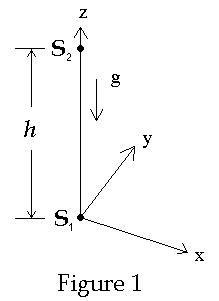Let the gravitational potential F(z) be defined such that F(0) = 0. Two pulses of light are emitted from S1 towards S2 spaced in time by the amount Dt as reckoned by coordinate time which is the term will use to the time as measured by observers in S1. Denote these events by E1(first pulse emitted from S1) and E2 (second pulse emitted from S1). Each pulse travels to S2 where they are recorded by observers in S2. Denote these events E’1 (first pulse received at S2) and E’2 (second pulse received at S2). The worldlines are drawn curved to allow for the possibility that the gravitational field may act on the light in an unknown way. In general light does not travel at a constant speed in a gravitational field but depends on the gravitational potential. A curved line represents a varying speed of light. The world lines of these light pulses are typically drawn in diagrams such as that shown in Figure 2 below.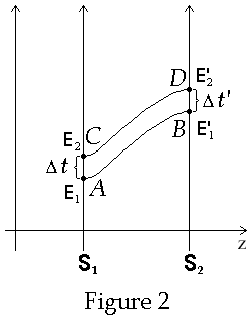Regardless of how the field acts on the light, the worldlines will be congruent. By this it is meant that the shape of the curves will be identical but merely translated upwards on the spacetime diagram. AC = BC as shown in Figure 2. However, it is argued, since it is known from experiment that
Dt > Dt’. Thus, Schild argues, AC ¹ BC and therefore the diagram is non-Euclidean, curved, spacetime. That the first derivation of gravitational redshift by Albert Einstein was in flat spacetime never seemed to bother Schild!
What’s wrong with Schild’s argument? First one needs to be careful when interpreting the statement "The frequency of light decreases..." Caution must be exercised when using "the" when discussing relativity. The frequency reckoned but which observer? Which clock is used to reckon this change? Secondly we note that when spacetime diagrams are drawn to illustrate Schild’s argument, they are always drawn without a scale on the time axes. This lack of a time scale leads one to make easily make an error. Each time axis represents the worldline of the clock located at the origin of its coordinate system. The clock in
S1 records the coordinate time in S1 whereas the clock in S2 records the coordinate time in S2. Since the two axes represent the time on different clocks which run at different rates the scale of the t-axis does not have the same scale as the t’-axis. The correct diagrams are shown in Figure 3 below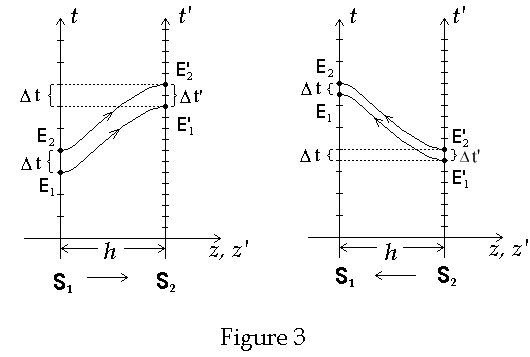Note that these are really two spacetime diagrams superimposed on top of each other, the difference between them being the origins are separated by the distance h, the spatial separation of the clock, i.e. S2 is located at a distance h higher in the gravitational field than S1.  Thus S2 is located at a higher gravitational potential than S2. In addition we add a scale to the times axes of both S1 and S2. Figure 3 shows a spacetime diagram of two light geodesics emitted from S1 towards S2. The t axis represents the worldline of clock C1 located in S1. The t’ axis represents the worldline of clock C2 located in S2. The proper time between two events on a particular clock is defined as the time recorded on a clock that passes through both events along a given worldline. The coordinate time of an event as recorded in S1, is found by projecting the event on the t’ axis onto the t axis (shown by dotted lines in Figure 3). The time interval is defined as the difference between the times of the events as recorded in S1. Let Dt be the proper time interval between events E1 and E2 as recorded in S1 and Dt’ be the proper time between events E’1and E’2 as recorded in S2. Then Dt is the proper time between E1 and E2 and Dt’ is the proper time reckoned by observers in S1 between E’1 and E’2. From the spacetime diagram in Figure 3, Dt is also the coordinate time as measured by observers in S1 between the events E’1 and E’2. However, due to the different time scales, while the emitter releases a pulse separate in time by one unit S1 receives the pulses separated in time by two units. As seen in Figure 3 Dt’ > Dt. In the diagram Dt = “1 unit of time” and Dt’ = “2 units of time.” In the diagrams we assume that C2 runs twice as fast as C1 and therefore Dt’ = 2Dt. This means that the frequency, at which the pulses leave S1, as recorded on C2, is twice the frequency at which the pulses arrive at S2, as recorded on C2. However, as reckoned by observers in S1 the rate of depart of the pulses from S1 is the same as the same rate that S2 receives them.

Schwarzschild Metric

In order to describe gravitational redshift consider a spherical symmetric massive body (e.g. planet, star, black hole etc.) whose gravitational field is described by the Schwarzschild metric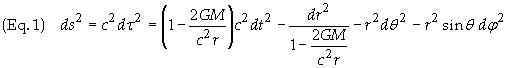ds is a scalar quantity which represents the invariant spacetime interval between two events in spacetime. For two events which have a spacelike spacetime separation the proper time between two events is related to ds by ds = c
2dt2. t is known as the coordinate time. r is the Schwarzschild radius, defined so that the circumference of a sphere at radius r is 2pr. It's also known as the coordinate radius or reduced circumference. q and f represent the typical spherical angles in a spherical coordinate system. The coordinate time t is defined as the time recorded by a clock located far away from the gravitating body. This time t is thus referred to sometimes as far-away time. Such a clock will be referred to below as a coordinate clock.
Consider two clocks Ce (Clock at emitter) and C
r (Clock at receiver) – which are of identical construction - located at the spatial coordinates Pe (re, qe , fe) and Pr (rr , qr , fr ). We wish to analyze the frequency of the light, locally, at both Pe and Pr . To do this we consider a sequence of pulses, which leaves Ce, and then analyze the rate at which these pulses arrive according to observers reading the time on Cr. We adjust the rate of the pulses emitted to be the frequency of the emitted light. We then determine the rate at which the pulses arrive at Cr and we will then have found the frequency of light, which arrives at Cr.
Let C
e emit two flashes of light, which then travel to Cr. Let each pulse of light travel along the same spatial path. Since the coordinate time t depends only on the spatial path the light takes, then the time it takes for each pulse to travel from Ce to Cr will be the same for each pulse.
Define

Dte = Coordinate time interval between the two signals which are emitted from Ce

Dtr = Coordinate time interval between the two signals which are received at Cr

It then follows that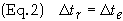Therefore the frequency of the light, as measured by any single observer, does not change as the light moves through the gravitational field!
According to Eq. (1) for two closely spaced pulses dt
r = dte . Let dt = dtr = dte = the coordinate time interval. The time interval recorded on C1 between the emission of two pulses is the proper time between the two pulses and will be denoted by dte . The time interval recorded on C2 between the reception of the same two pulses is the proper time between the two pulses arriving at C2 and denoted dtr.  These intervals are related to the proper time intervals through the metric. For simplicity let dr = dq = df = 0 (i.e. the clocks are at rest).  Substituting these into the Schwarzschild metric gives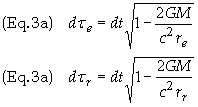Upon rearranging terms we get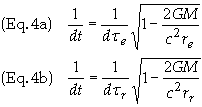Note that frequencies are related to time intervals by f = 1/dt. Define the following frequencies

f = frequency as measured on far-away clock

fe = frequency as measured on Ce

fr = frequency as measured on Cr

Then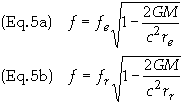Equating Eq. 3b by Eq. 3a becomes upon rearranging terms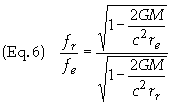This is the relation for gravitational redshift. Eq. 5 shows that when re ¹ rr then fe ¹ fr. Consider now that the light consists of photons. Multiply Eq. (4) through by Plank's constant h, noting that the energy of a photon is E = hf, to get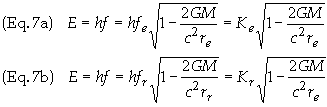E is then the energy of photon as measured by a far-away observer. Ke and Kr are the energies of the photons when measured by devices collocated with the clocks at Pe and at Pr respectively. These energies may be thought of as the kinetic energies of the photons. Eq. 5 can be rearranged to give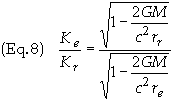Eq. 6 can be simplified by using the approximation (1+d)n » 1 + nd,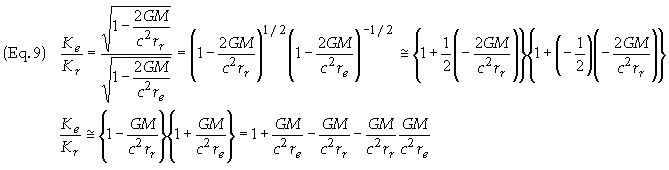neglecting second order terms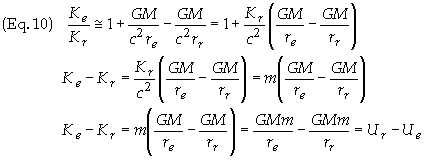whew m = Kr /c2 = gravitational mass of photon as measured by observer at r = re. U º -GMm/r = Gravitationnal Potential Energy of photon and K = Kinetic Energy of Photon (energy of photon measured locally). Then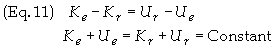Define E = K + U = Kinetic Energy + Potential Energy = total inertial energy. Therefore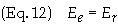which is simply the expression for the conservation of energy. Since this holds for any r it follows that E = constant along the photon's trajectory. I.e. The total energy of a photon moving through a gravitational field is constant.

 A. Schild, Monist 40, 20 (1962) Evidence for Gravitational Theories, edited by C. Moller (Academic, New York, 1962); Conference Internationalesur les theories relativistes de la gravitation, edited by L. Infeld (Pergamon, Oxford. 1964).
 Does a gravitational red shift necessarily imply that spacetime is curved?, by G.E. Marsh and C. Nissim-Sabat. Am. J. Phys. Vol. 43, No. 3, March (1975)

Hosted by www.Geocities.ws